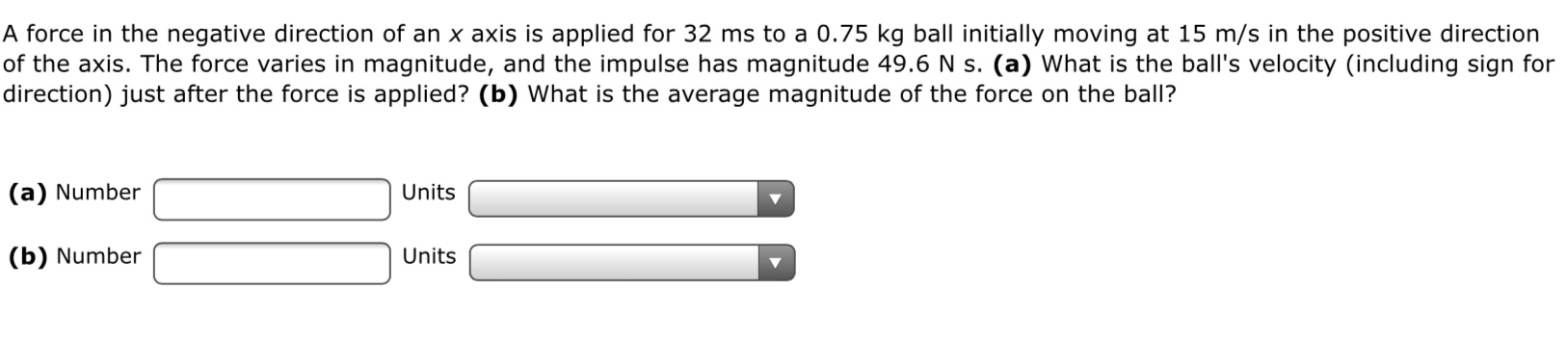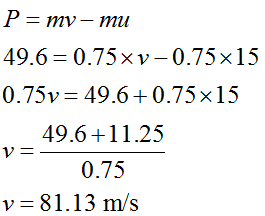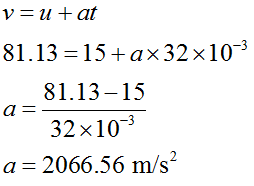# A force in the negative direction of an x axis is applied for 32 ms to a 0.75 kg ball initially moving at 15 m/s in the positive directionof the axis. The force varies in magnitude, and the impulse has magnitude 49.6 N s. (a) What is the ball's velocity (including sign fordirection) just after the force is applied? (b) What is the average magnitude of the force on the ball?(a) NumberUnits(b) NumberUnits

Question
1 viewshelp_outlineImage TranscriptioncloseA force in the negative direction of an x axis is applied for 32 ms to a 0.75 kg ball initially moving at 15 m/s in the positive direction of the axis. The force varies in magnitude, and the impulse has magnitude 49.6 N s. (a) What is the ball's velocity (including sign for direction) just after the force is applied? (b) What is the average magnitude of the force on the ball? (a) Number Units (b) Number Units fullscreen
check_circle

Step 1

Given:

Direction of force is in negative x direction.

Implementation of force for time (t) = 32 ms.

Mass of the ball (m) = 0.75 kg.

Initial velocity of ball (u) = 15 m/s.  (+x direction)

Magnitude of impulse (P) = 49.6 Ns.

Step 2

Part a)

The impulse is given as,Step 3

Part b)

The acceleration of ball during the application of force,...

### Want to see the full answer?

See Solution

#### Want to see this answer and more?

Solutions are written by subject experts who are available 24/7. Questions are typically answered within 1 hour.*

See Solution
*Response times may vary by subject and question.
Tagged in